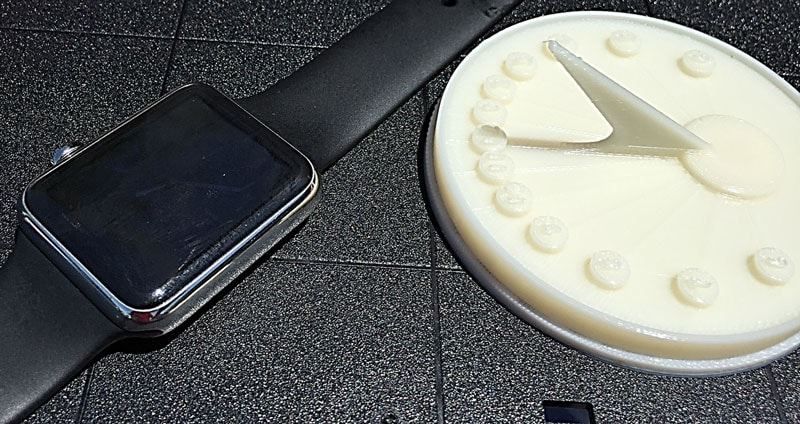## 3D Printing the 4th Dimension – GISHWHES 2015 Scavenger HuntGISHWHES is a huge international scavenger hunt. Every year teams around the globe comb through the list of 215 tasks and pick as many as possible that their team can do.  Last year they introduced 3D Printing as a task, and we helped a team 3D Print a quill pen. That was a lot of fun, so when this year’s list included an item on 3D printing, we jumped at the chance to be involved.

The item was:

110: VIDEO. Use a cutting edge 3D printer to 3D print your representation of the 4th dimension.62 POINTS

Being engineers we said “4th Dimension?  Time.”  Then it became a choice between the way mass distorts the space-time continuum or some sort of clock’ish thing.  The distortion thing seemed difficult so we focused on a clock.  Being that we were constrained on budget and time we decided to do a sundial.

The result can be seen here in this YouTube video.

It was a fun project and the team spent a bit of time in the 112F sunshine trying it out.  We can’t wait to see what we will get to do for the 2016 scavenger hunt.

# Making the Model

A couple of people have asked if we downloaded the solid model for the sundial or if we made it. We actually made it. After a little bit of research we found that making a simple horizontal sundial like this one is very easy. Here are the steps we took:

## Get Geometry Values

So it turns out that the angle of each hour line is determined by the latitude of where the dial will go. The angle of the pointy thing, called a gnomon, is also the latitude.  So for Tempe, AZ that is 33.4294°.That gets applied to the equation:

angle(h) = arctan(sin(L*tan(15° · h))

h = integer of the hour, 6 am to 6 pm
L = latitude

I plopped that into Excel:

=ABS(DEGREES(ATAN(SIN(RADIANS(\$C\$3))*TAN(RADIANS(15)*B7))))

and got the following:

 Latitude 33.4294 Hour Angle 6 90.00 7 64.06 8 43.66 9 28.85 10 17.64 11 8.40 12 0.00

## Build the Solid Model

The next step is to build the model. I used SolidEdge because I know it real well and was able to knock it out quickly.  But all CAD tools would be the same:

1. Pick a center point.
2. Add lines as rays from that using the angles in the table above for each hour.
3. Design the shape of your sundial to look cool. I did a simple circle .
4. Mark the hours using the sketch. I raised up thin rectangles.
5. Model the gnomon using the latitude as the angle.  Make this as fancy or simple as you want.
6. Add whatever doo-dads you want.
7. Label the hours if you want.
8. Save to STL

And the final solid model looked like this:We sent this to the printer as shown in the video, and got a sundial.